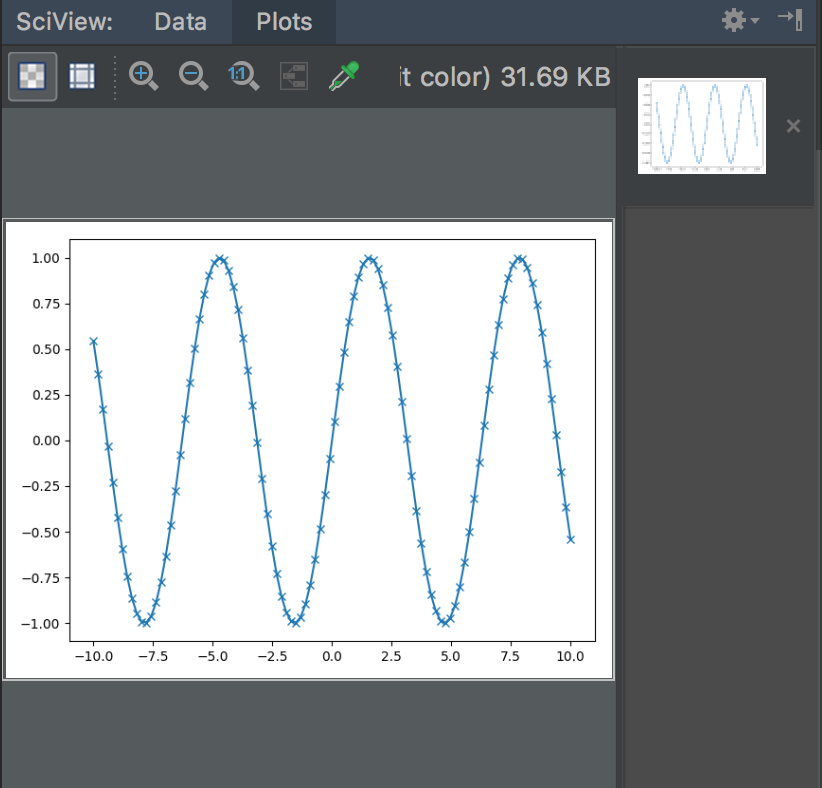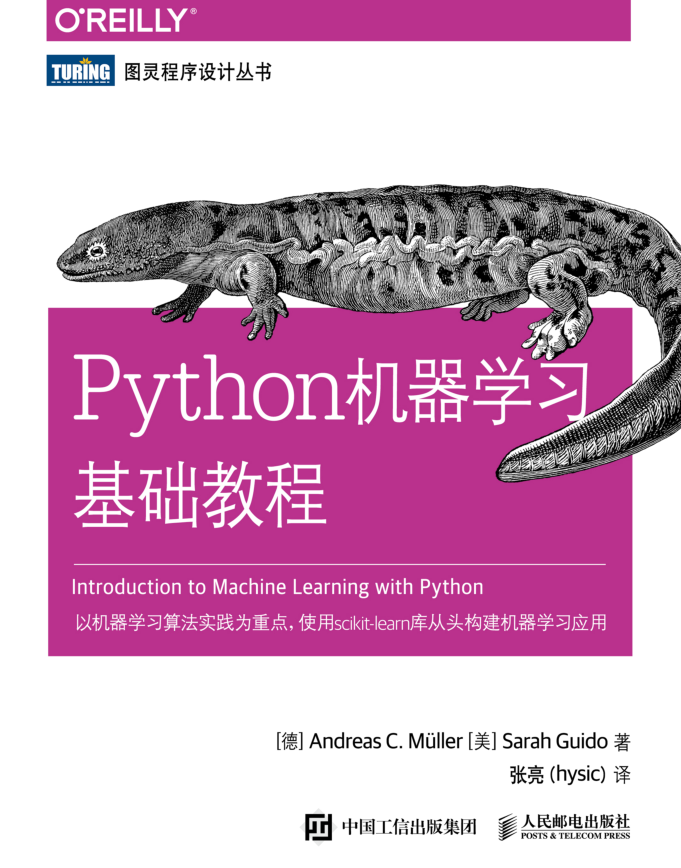• python机器学习入门-用鸢尾花data建立python机器学习，本次运行Python版本为3.6.2，且已安装相关python库
• 数据集介绍 总共包含150行数据 每一行数据由 4 个特征值及一个目标值组成。 4 个特征值分别为：萼片长度、萼片宽度、花瓣长度、花瓣宽度 目标值为三种不同类别的鸢尾花，分别为： Iris Setosa、Iris Versicolour、...
• 开始学习机器学习基础，在此留下学习心得与自己的理解。 啥也不说，先看一下鸢尾花啥样 原创文章 21获赞 17访问量 1688 关注 私信 展开阅读全文 作者：会飞的小猪崽儿
• ## python机器学习入门到精通(一)

万次阅读 多人点赞 2018-06-09 12:29:53
机器学习概论 机器学习，顾名思义，它是人工智能、统计学、计算机科学交叉的研究领域，也被称作预测分析或统计学习。近年来，由于人工智能的火爆，机器学习方法已经应用到日常生活的方方面面。贴近我们生活的就有，...
机器学习概论
机器学习，顾名思义，它是人工智能、统计学、计算机科学交叉的研究领域，也被称作预测分析或统计学习。近年来，由于人工智能的火爆，机器学习方法已经应用到日常生活的方方面面。贴近我们生活的就有，系统自动推荐用户的喜好，买什么物品，点什么食物，浏览新闻时，给你推送什么类型的文章，甚至于我们登录淘宝购买物品，微博观看别人的微博时，这些每一部分都可能包含多种机器学习模型。当然，机器学习也在科研方面也有建树，比如DNA分析，天文学的研究，微观粒子等。同时，要想学好机器学习，数学的基础也是必须的。  机器学算法是将决策过程自动化的算法，而这些决策是从已知示例中泛化得出来的，而这种方法被称作监督学习(supervised learning)。 用户将成对的输入和预期输出提供给算法，然后算法会找到一种方法，根据给定输入给出预期输出。从输入/输出对中进行学习的机器学习算法叫作监督学习算法，因为每个用于算法学习的样例都对应一个预期输出，就像老师教导学生在督算着算法。在生活中，监督机器学习任务大多应用于：
识别信封上手写的邮政编码, 输入扫描的手写数字，预期输出是邮政编码中的实际数字。想要创建用于构建机器学习模型的数据集，需要收集许多信封，然后可以自己阅读邮政编码，将数字保存为预期输出。基于医学影像判断肿瘤是否为良性, 这里的输入是影像，输出是肿瘤是否为良性。创建用于构建模型的数据集，需要一个医学影像数据库，需要查看所有影像，然后判断哪些肿瘤是良性和不是良性的。检测信用卡交易中的诈骗行为， 输入是信用卡交易记录，输出是该交易记录是否可能为诈骗，收集数据集意味着需要保存所有的交易，并记录用户是否上报过任何诈骗交易。确定一系列博客文章的主题，拥有许多文本数据，对其进行汇总，并找到其中共同的主题。将客户分成具有相似偏好的群组，给定一组客户记录， 需要找出哪些客户比较相似，并判断能否根据相似偏好对这些客户进行分组。检测网站的异常访问模式，识别网站的滥用或bug，找到异常的访问模式。
scikit-learn 是一个开源项目，而scikit-learn依赖于另外两个python包：NumPy和SciPy, matplotlib、IPython和 Jupyter Notebook可以帮助我们进行绘图和进行交互式开发。  NumPy是python科学计算的基础包之一，NumPy数组是基本数据机构。它的功能包括多维数组、高级数学函数以及伪随机数生成器。Numpy的核心功能是ndarray类，即多维数组。数组的所有元素必须是同一类型，如：
import numpy as np
x = np.array([[1,2,3],[4,5,6]])
print("x:\n{}".format(x))
输出：
x:[[1 2 3]
[4 5 6]]
SciPy是python中用于科学计算的函数集合。它具有线性代数高级程序、数学函数优化、信号处理、特殊数学函数和统计分布等多项功能。SciPy中最重要的是scipy.sparse，它可以给出稀疏矩阵，而稀疏矩阵是scikit-learn中数据的另一种表示方法。如果想保存一个大部分元素都是0的二维数组，就可以使用稀疏矩阵：
from scipy import sparse
# 创建一个二维NumPy数组，对角线为1，其余都为0
eye = np.eye(4)
print("NumPy array:\n{}".format(eye))
输出：
NumPy array:
[[1. 0. 0. 0.]
[0. 1. 0. 0.]
[0. 0. 1. 0.]
[0. 0. 0. 1.]]
# 将NumPy数组转换为CSR格式的SciPy稀疏矩阵
# 只保存非零元素
sparse_matrix = sparse.csr_matrix(eye)
print("\nSciPy sparse CSR matrix:\n{}".format(sparse_matrix))
输出：
SciPy sparse CSR matrix:
(0,0)  1.0
(1,1)  1.0
(2,2)  1.0
(3,3)  1.0
通常来讲，创建稀疏数据的稠密表示是不可能的(因为太耗费内存)，所以我们需要直接创建其稀疏表示。我们再用COO格式创建同一稀疏矩阵：
data = np.ones(4)
row_indices = np.arange(4)
col_indices = np.arrange(4)
eye_coo = sparse.coo_matrix((data, (row_indices, col_indices)))
print("COO representation:\n{}".format(eye_coo))

输出：
COO representation:
(0,0)  1.0
(1,1)  1.0
(2,2)  1.0
(3,3)  1.0
matplotlib是python主要的科学会图库，功能为生成可发布的可视化内容，如折线图、直方图、散点图等。在Jupyter Notebook中，你可以使用%matplotlib notebook 和%matplotlib inline命令，将图像直接显示在浏览器中。举个例子方便理解下：
import numpy as np
import matplotlib.pyplot as plt
在 -10和10之间生成一个数列，共100个数
x = np.linspace(-10,10,100)
用正弦函数创建第二个数组
y = np.sin(x)
plot函数绘制一个数组关于另一个数组的折线图
plt.plot(x, y, marker="x")
plt.show()pandas是用于处理和分析数据的python库。它是基于一种叫作DataFrame的数据结构，这种数据结构模仿了R语言中的DataFrame。而pandas DateFrame是一张表格，类似于Excel表格，所以pandas中包含大量用于修改表格和操作表格的方法，使能够像SQL一样对表格进行查询和链接。NumPy要求数组中的所有元素类型必须完全相同，而pandas每一列数据的类型可以互不相同(比如整型、日期、浮点数和字符串)。pandas还可以从许多文件格式和数据库中提取数据，如SQL、Excel文件和逗号分隔值(csv)文件。  我们利用字典创建DataFrame:
import pandas as pd
from IPython.display import display
# 创建关于人的简单数据集
data = {'Name':["James", "Wade", "Curry", "Ken"],
'Location': ["Cleveland", "SFC", "LSA", "NY"],
'Age':[33,35,27,16]}
data_pandas = pd.DataFrame(data)
# IPython.display可以在Jupyter Notebook中打印出美观的DataFrame
display(data_pandas)
如果想要查询也很简单，比如：
#选择年来大于30的所有行
display(data_pandas[data_pandas.Age > 30]
以上便是机器学习中，python需要的几个库，让我们分析数据变得更轻松，更能解决各种问题。机器学习和数据分析本质上都是迭代过程，由数据驱动分析，这些过程必须要由快速迭代和易于交互的工具。而机器学习中，最重要的部分就是理解我们在处理的数据，以及这些数据与任务之间的关系，并选择算法。所以在我们构建模型之前，我们必须要理解数据集的内容。
展开全文人工智能
• 燕大软件机器学习实验报告，六个模型学习，实验报告下载了就能用，很方便，下载就行，直接用，燕大嘞。鸢尾花，波士顿，猫狗分类什么的
• python机器学习基础教程 思维导图 第1，第2章，逐步更新 使用Xmind打开 帮助梳理巩固知识点 纯手打 供交流学习使用
• 机器学习概述、特征工程、knn、朴素贝叶斯、决策树、随机森林、线性回归、非线性回归、逻辑斯蒂回归、k-means聚类算法
• ## Python机器学习基础教程

千次阅读 热门讨论 2019-12-22 15:00:23
Python机器学习教程 第一章：对于机器学习，我们选择了Python 第二章：了解SciPy、Pandas
Python机器学习教程
第一章：对于机器学习，我们选择了Python
第二章：了解SciPy、Pandas
持续更新 ... ...展开全文人工智能
• description: 《Python机器学习基础教程》的第一章笔记，书中用到的相关代码见github:https://github.com/amueller/introduction_to_ml_with_python， 笔记中不会记录。

description: 《Python机器学习基础教程》的第一章笔记，书中用到的相关代码见github:https://github.com/amueller/introduction_to_ml_with_python ，笔记中不会记录。
为何选择机器学习
人为制订决策规则主要有两个缺点：
做决策所需要的逻辑只适用于单一领域和单项任务。任务哪怕稍有变化，都可能需要重写整个系统。想要制订规则，需要对人类专家的决策过程有很深刻的理解。
机器学习能够解决的问题
监督学习 从输入/输出对中进行学习的机器学习算法叫作监督学习算法（supervised learning algorithm），用于算法学习的样例都对应一个预期输出， 好像有一个“老师”在监督着算法。无监督学习 只有输入数据是已知的，没有为算法提供输出数据。虽然这种算法有许多成功的应用，但理解和评估这些算法往往更加困难。样本&特征 通常来说，将数据想象成表格是很有用的，这里的每个实体或每一行被称为一个样本（sample）或数据点，而每一列（用来描述这些实体的属性）则被称为特征（feature）。
熟悉任务和数据
我想要回答的问题是什么？已经收集到的数据能够回答这个问题吗？要将我的问题表示成机器学习问题，用哪种方法最好？我收集的数据是否足够表达我想要解决的问题？我提取了数据的哪些特征？这些特征能否实现正确的预测？如何衡量应用是否成功？机器学习解决方案与我的研究或商业产品中的其他部分是如何相互影响的？
为何选择Python
机器学习和数据分析本质上都是迭代过程，由数据驱动分析。这些过程必须要有快速迭代和易于交互的工具。
scikit-learn
它包含许多目前最先进的机器学习算法，每个算法都有详细的 文档 scikit-learn 也可以与其他大量 Python 科学计算工具一起使用。浏览 scikit-learn 用户指南 和 API 文档，里面给出了每个算法的更多细节和更多选项。
必要的库和工具
如果不熟悉 NumPy 或 matplotlib，推荐阅读 SciPy 讲稿 的第 1 章
Jupyter Notebook
书中所有内容都是以 Jupyter Notebook 的形式进行编写的。所有代码示例都可以在 GitHub 下载 。
NumPy
它的功能包括多维数组、高级数学函数（比如线性代数运算和傅里叶变换），以及伪随机数生成器。在 scikit-learn 中， NumPy 数组是基本数据结构，你用到的所有数据都必须转换成 NumPy 数组。NumPy 的核心功能是 ndarray 类，即多维（n 维）数组。数组的所有元素必须是同一类型。
SciPy
用于科学计算的函数集合。它具有线性代数高级程序、数学函数优化、信号处理、特殊数学函数和统计分布等多项功能。对我们来说， SciPy 中最重要的是 scipy.sparse：它可以给出稀疏矩阵（sparse matrice），稀疏矩阵是 scikit-learn 中数据的另一种表示方法。如果想保存一个大部分元素都是 0 的二维数组，就可以使用稀疏矩阵。关于 SciPy 稀疏矩阵的更多内容可查阅SciPy 讲稿 。
matplotlib
在 Jupyter Notebook 中， 可以使用 %matplotlib notebook 和 %matplotlib inline 命令，将图像直接显示在浏览器中。我们推荐使用%matplotlib notebook 命令，它可以提供交互环境（虽然在本书中使用的是%matplotlib inline）。
pandas
基于一种叫作 DataFrame 的数据结构。一个 pandas DataFrame 是一张表格，类似于 Excel 表格。 pandas 中包含大量用于修改表格和操作表格的方法，尤其是可以像 SQL 一样对表格进行查询和连接。它可以从许多文件格式和数据库中提取数据，如SQL、 Excel 文件和逗号分隔值（CSV）文件。
mglearn
书的附加代码可以在 GitHub 下载 附加代码不仅包括书中的所有示例，还包括 mglearn 库。 这是为本书编写的实用函数库，以免将代码清单与绘图和数据加载的细节混在一起。书会频繁使用 NumPy、 matplotlib 和 pandas。所有代码都默认导入了这些库：
import numpy as np
import matplotlib.pyplot as plt
import pandas as pd
import mglearn

Python 2与Python 3的对比
3>2
本书用到的版本
见代码
import sys
print("Python version: {}".format(sys.version))
import pandas as pd
print("pandas version: {}".format(pd.__version__))
import matplotlib
print("matplotlib version: {}".format(matplotlib.__version__))
import numpy as np
print("NumPy version: {}".format(np.__version__))
import scipy as sp
print("SciPy version: {}".format(sp.__version__))
import IPython
print("IPython version: {}".format(IPython.__version__))
import sklearn
print("scikit-learn version: {}".format(sklearn.__version__))

第一个应用——鸢尾花分类
监督学习->分类->三分类问题
初识数据
本例中用到了鸢尾花（Iris）数据集，这是机器学习和统计学中一个经典的数据集。它包含在 scikit-learn 的 datasets 模块中。我们可以调用 load_iris 函数来加载数据。
衡量模型是否成功： 训练数据与测试数据
模型会一直记住整个训练集，所以对于训练集中的任何数据点总会预测正确的标签。这种“记忆”无法告诉我们模型的泛化（generalize）能力如何（换句话说，在新数据上能否正确预测）。用新数据来评估模型的性能->将收集好的带标签数据（此例为 150 朵花的测量数据）分成两份->一份用于构建机器学习模型，叫作训练数据（training data）或训练集（training set），其余用来评估模型性能，叫作测试数据（test data）、测试集（test set）或留出集（hold-out set）。

scikit-learn 中的 train_test_split 函数可以打乱数据集并进行拆分。这个函数将 75% 的行数据及对应标签作为训练集，剩下 25% 的数据及其标签作为测试集。（训练集与测试集的分配比例可以是随意的，但使用 25% 的数据作为测试集是很好的经验法则）

scikit-learn 中的数据通常用大写的 X 表示，而标签用小写的 y 表示。这是受到了数学标准公式 f(x)=y 的启发，其中 x 是函数的输入， y 是输出。我们用大写的 X 是因为数据是一个二维数组（矩阵），用小写的 y 是因为目标是一个一维数组（向量），这也是数学中的约定。

为了确保多次运行同一函数能够得到相同的输出，这里利用 random_state 参数指定了随机数生成器的种子。这样函数输出就是固定不变的，所以这行代码的输出始终相同。本书用到随机过程时，都会用这种方法指定 random_state。

要事第一： 观察数据
在构建机器学习模型之前，通常最好检查一下数据。

检查数据也是发现异常值和特殊值的好方法

检查数据的最佳方法之一就是将其可视化，有两种方法： （1）绘制散点图：数据散点图将一个特征作为 x 轴，另一个特征作为 y 轴。 （2）绘制散点图矩阵：可以两两查看所有的特征。

pandas 有一个绘制散点图矩阵的函数，叫作 scatter_matrix

构建第一个模型： k近邻算法
k 近邻算法中 k 的含义是，我们可以考虑训练集中与新数据点最近的任意 k 个邻居（比如 说，距离最近的 3 个或 5 个邻居），而不是只考虑最近的那一个。然后，我们可以用这些 邻居中数量最多的类别做出预测。

现在我们只考虑一个邻居的情况

scikit-learn 中所有的机器学习模型都在各自的类中实现，这些类被称为 Estimator类（需实例化为对象才可使用该模型）。

knn 对象对算法进行了封装，既包括用训练数据构建模型的算法，也包括对新数据点进行 预测的算法

想要基于训练集来构建模型，需要调用 knn 对象的 fit 方法。
做出预测
发现新花，将这朵花的测量数据转换为二维 NumPy 数组的一行，调用 knn 对象的 predict 方法来进行预测。产生预测结果，但由于并不知道这个样本的实际品种，故要进行结果评估。
评估模型
需要用到之前创建的测试集，可以对测试数据中的每朵鸢尾花进行预测，并将预测结果与标签（已知的品种）进行对比。通过计算精度（accuracy）来衡量模型的优劣，精度就是品种预测正确的花所占的比例。对于这个模型来说，测试集的精度约为 0.97。
小结与展望
学接口，学调参orz
展开全文笔记
• python机器学习入门-用鸢尾花data建立python机器学习，本次运行Python版本为3.6.2，且已安装相关python库
• 随着信息化的不断发展，人工智能、机器学习等词语越来越被人们所熟知，而他们也渐渐地成了这个时代的弄潮儿，走在了信息时代的前端。从本篇博客开始，小编将带领大家一起走进人工智能之机器学习部分，让深度学习不再...
       当今世界已经进入了大数据的时代。随着信息化的不断发展，人工智能、机器学习等词语越来越被人们所熟知，而他们也渐渐地成了这个时代的弄潮儿，走在了信息时代的前端。从本篇博客开始，小编将带领大家一起走进人工智能之机器学习部分，让深度学习不再成为困难。同时，相关的文章将会发布在我的博客专栏《Python从入门到深度学习》，欢迎大家关注~
《Python从入门到深度学习》这一系列的博客是小编深思熟虑之后决定写的，目的是方便大家共同学习，也是为了巩固自己的知识。本专栏以代码为主，相关算法的原理讲解会在本专栏更新完之后，单独的列出一个新的算法专栏进行讲解，敬请期待。为了方便共同学习，小编在代码中列出了详细的注释。

目录
一、Python机器学习基础之Python的基本语法（一）
二、Python机器学习基础之Python的基本语法（二）
三、Python机器学习基础之Numpy库的使用
四、Python机器学习基础之Pandas库的使用
五、Python机器学习基础之Matplotlib库的使用
六、Sklearn库和TensorFlow框架
七、Python两种方式加载文件内容

声明：代码的运行环境为Python3。Python3与Python2在一些细节上会有所不同，希望广大读者注意。下面开始我们的第一讲：Python机器学习基础之Python的基本语法（一）。

【代码】
'''
机器学习基础之Python的基本语法
'''

# 打印数据到控制台：python2中是不需要括号的；python3需要带括号
print("Hello World!!")  # 值得一提的是，代码结尾的;是可有可无的
# 同时打印多个变量，各个变量之间用,隔开
a = "我是:"
b = "象在舞"
print(a, b)
# python3中支持了Unicode（utf-8）字符串
姓名 = '象在舞'
print(姓名)

# 注解的使用：单行注释采用#开头；多行注释使用三个单引号'''或三个双引号"""表示

# 数据类型：1、Python 中的变量赋值不需要类型声明；2、Python中，所有标识符可以包括英文、数字以及下划线(_)，但不能以数字开头，区分大小写；
# 3、以下划线开头的标识符有特殊意义。如：_foo 不能直接访问，需通过类提供的接口进行访问；4、在 python 中，变量是没有类型的，如：a=[1,2,3];a="Runoob"
# [1,2,3] 是 List 类型，"Runoob" 是 String 类型，而变量 a 是没有类型，它仅仅是一个对象的引用（一个指针），可以指向 List 类型对象，也可以指向String类型对象;
# Python3有六个标准的数据类型：Number（数字）;String（字符串）;List（列表）;Tuple（元组）;Dictionary（字典）;Set（集合，Python3新加的）

# 数字：Python3支持三种不同的数字类型：int（有符号整型）；float（浮点型）；complex（复数：由实数和虚数构成，用a + bj,或complex(a,b)来表示）
# long（长整型，注：在Python3里被去除了)
a = 100
print(a)
b = 1.23
print(b)
c = 10 + 2j  # 复数(实数+虚数)
print(c)

# 字符串：使用引号( ' )、双引号( " )、三引号( ''' 或 """ )来表示字符串，其中三引号可以由多行组成
a = 'xzw'
b = "我是象在舞"
c = '''我是象在舞,
来自上海'''
print(a, b, '\n', c)  # 中间用空格间隔

str = 'Hello World!'
print(str)
print(str)         # 输出字符串中的第一个字符
print(str[2:5])       # 输出字符串中第三个至第五个(不包含第6个)之间的字符串
print(str[2:])        # 输出从第三个字符开始的字符串
print(str * 2)        # 输出字符串两次
print(str + "I am xzw.")   # 输出连接的字符串
# print(str+1)         # 错误，不可以是非字符串类型(和java不一样)
# 这种方法效率低，python中字符串是不可变的类型，使用 + 连接两个字符串时会生成一个新的字符串，生成新的字符串，就需要重新申请内存，当连
# 续相加的字符串很多时(a+b+c+d+e+f+...) ，效率低下就是必然的了
print('a' + 'b' + 'c')
# 略复杂，但对多个字符进行连接时效率高，只会有一次内存的申请。如果是对list的字符进行连接的时候，这种方法是首选
listStr = ['a', 'b', 'c']
s = ''.join(listStr)
print(s)
# 字符串格式化，这种方法非常常用
print('%s%s%s' % ('a', 'b', 'c'))
print( 'Hello: %s %s %s' % ('xzw', 100, 22.3) )
# 多个变量同时赋值
a, b, c = 1, 2.2, "xzw"
print(a, b, c)

# 列表：List（列表）是Python中使用最频繁的数据类型，列表支持字符，数字，字符串，还可以包含列表（即嵌套）
list = ['xzw', 786, 2.23, 'Eric', 70.2]
tinylist = [123, 'Eric']
print(list)               # 输出完整列表
list = 'aaa'
print(list)            # 输出列表的第一个元素
print(list[1:3])          # 输出第二个至第三个的元素，不包括第四个
print(list[2:])           # 输出从第三个开始至列表末尾的所有元素
print(tinylist * 2)       # 列表元素重复两次
print(list + tinylist)    # 将两个列表合并

# 元组：组是另一种数据类型，类似于List（列表）。元组用"()"标识。内部元素用逗号隔开。但是元组不能二次赋值，相当于只读列表。
tuple = ( 'xzw', 786 , 2.23, 'Eric', 70.2 )
tinytuple = (123, 'Eric')
print(tuple)               # 输出完整元组
# tuple='aaa'           # 错误
print(tuple)            # 输出元组的第一个元素
print(tuple[1:3])          # 输出第二个至第三个的元素
print(tuple[2:])           # 输出从第三个开始至列表末尾的所有元素
print(tinytuple * 2)       # 输出元组两次
print(tuple + tinytuple)   # 打印组合的元组

# 字典
dict = {}
dict['xzw'] = "This is xzw"
dict = "This is two"
tinydict = {'name': 'xzw', 'code':6734, 'dept': 'sales'}
print(dict['xzw'])          # 输出键为'one' 的值
print(dict)              # 输出键为 2 的值
print(tinydict)             # 输出完整的字典
print(tinydict.keys())      # 输出所有键
print(tinydict.values())    # 输出所有值

# Set：Python3新加入的
student = {'Tom', 'Jim', 'Mary', 'Tom', 'Jack', 'Rose'}
print(student)   #重复的元素被自动去掉
if('Rose' in student) :
print('Rose 在集合中')
else :
print('Rose 不在集合中')
# set可以进行集合运算
a = set('abcde')
b = set('abcfg')
print(a)
print(b)
print(a - b)     # a和b的差集
print(a | b)     # a和b的并集
print(a & b)     # a和b的交集
print(a ^ b)     # a和b中不同时存在的元素

# bytes类型：Python3新加入的
b = b'xzw'
print(b)     #打印出的是ascii码
print(type(b))  #查看类型
s = b.decode()  #转换为字符串
print(s)
b1 = s.encode() #转换为bytes类型
print(b1)

# 可变与不可变：在 python 中，string, tuple, 和 number 是不可更改的对象，而 list,dict 等则是可以修改的对象
# 不可变类型：变量赋值 a=5 后再赋值 a=10，这里实际是新生成一个 int 值对象 10，再让 a 指向它，而 5 被丢弃，不是改变a的值，相当于新生成了a
# 可变类型：变量赋值 list=[1,2,3,4] 后再赋值 list=5 则是将llist的第三个元素值更改， list本身没有动，只是其内部的一部分值被修改了

# 运算符
print(2 ** 3)  # 8 求幂
print(10 // 3)  # 3 求整数商
print(1 / 2)  # 0.5，和其他语言有所不同

a = 100
a += 1  # 没有a++
print(a)

# 关系运算符： > < == != ( <> 在Python3中不支持) >= <=
# 逻辑运算符：0为假，非0为真
a = 10
b = 20
if (a and b):
print("1 - 变量 a 和 b 都为 true")
else:
print("1 - 变量 a 和 b 有一个不为 true")

if (a or b):
print("2 - 变量 a 和 b 都为 true，或其中一个变量为 true")
else:
print("2 - 变量 a 和 b 都不为 true")

# 修改变量 a 的值
a = 0
if (a and b):
print("3 - 变量 a 和 b 都为 true")
else:
print("3 - 变量 a 和 b 有一个不为 true")

if (a or b):
print("4 - 变量 a 和 b 都为 true，或其中一个变量为 true")
else:
print("4 - 变量 a 和 b 都不为 true")

if not (a and b):
print("5 - 变量 a 和 b 都为 false，或其中一个变量为 false")
else:
print("5 - 变量 a 和 b 都为 true")

# 成员运算符：in、not in
a = 1
b = 20
list = [1, 2, 3, 4, 5];

if (a in list):
print("1 - 变量 a 在给定的列表中 list 中")
else:
print("1 - 变量 a 不在给定的列表中 list 中")

if (b not in list):
print("2 - 变量 b 不在给定的列表中 list 中")
else:
print("2 - 变量 b 在给定的列表中 list 中")

# 身份运算符
# is 是判断两个标识符是不是引用自一个对象，x is y 类似 id(x) == id(y)
# is 与 == 区别：is 用于判断两个变量引用对象是否为同一个， == 用于判断引用变量的值是否相等
a = 20
b = 20
print(a is b)
print(a is not b)
# 修改变量 b 的值
b = 30
print(a is b)
print(a is not b)
# is在Python命令行中比较特殊，为了提高内存利用效率对于一些简单的对象，如一些数值较小的int对象，python采取重用对象内存的办法，如指向a = 2，b = 2
# 时，由于2作为简单的int类型且数值小，python不会两次为其分配内存，而是只分配一次，然后将a与b同时指向已分配的对象
# c = 20
# d = 20
# c is d
# True
# 如但果赋值的不是2而是较大的数值，情况就不一样了：
# c = 5555
# d = 5555
# c is d
# False

# 条件：python并不支持switch语句
num = 5
if num == 3:
print('boss')
elif num == 2:
print('user')
elif num == 1:
print('worker')
elif num < 0:
print('error')
else:

# 代码组：Python与其他语言最大区别是，Python代码块不使用大括号，Python最具特色的就是用缩进来写模块，缩进相同的一组语句构成一个代码块，我们称之代码组
# 像if、while 、def和class等首行以关键字开始，以冒号结束，该行之后的一行或多行代码构成"代码组"
# 我们将首行及后面的代码组称为一个"子句"(clause)缩进的空白数量是可变的，但是所有代码块语句必须包含相同的缩进空白数量，这个必须严格执行!!!
# 例如：
# if expression:
#     suite  # 代码组
# elif expression:
#     suite
# else:
#     suite
# 例如：(注意：没有严格缩进，在执行时会报错)。通过coding属性支持中文(在不支持中文时，加上该句)
# 下面代码会报缩进错误IndentationError
# # !/usr/bin/python
# # -*- coding: UTF-8 -*-
# if True:
#     print("aaa")
#     print("True")
# else:
#     print("bbb")
# print("False")  # 注意缩进，只要对齐就行，至于用空格还是用tab对齐没区别
# 注意：有些环境下，tab和空格不可以混用，有些环境下可以，因此建议在每个缩进层统一使用。'单个制表符'、'两个空格'或'四个空格'，尽量不要混用，以避免不必要的麻烦

# while
count = 0
while count < 10:
# while (count < 10) :  #也可以
print('The count is:', count)
count = count + 1
# while … else …：循环条件为false时，执行else语句块，只执行一次
count = 0
while count < 5:
print(count, " is  less than 5")
count = count + 1
else:
print(count, " is not less than 5")

# continue
i = 1
while i < 10:
i += 1
if i % 2 != 0:  # 非双数时跳过输出
continue
print(i)  # 输出双数2、4、6、8、10

# break
i = 1
while True:
print(i)
i += 1
if i > 10:  # 当i大于10时跳出循环
break

# for
for letter in 'Python3':
print('当前字母 :', letter)

names = ['xzw', 'Eric', 'Josie']
for name in names:
print('当前姓名 :', name)

# 通过序列索引迭代
names = ['xzw', 'Eric', 'Josie']
for index in range(len(names)):
# for index in range(3): #效果相同，即(1,2,3)
print('当前姓名 :', names[index])

# for ...else
for num in range(2, 10):  # 迭代 2 到 10 之间的数字
for i in range(2, num):  # 根据因子迭代
if num % i == 0:  # 确定第一个因子
j = num / i  # 计算第二个因子
print('%d 等于 %d * %d' % (num, i, j))
break  # 跳出当前循环
else:  # 循环的 else 部分
print(num, '是一个质数')

# pass:pass语句不做任何事情，一般用做占位
for letter in 'Python':
if letter == 'h':
pass
print('这是 pass 块')
else:
print('当前字母 :', letter)

# 字符串中插入变量：
# 方法一：
name = 'xzw'
sayhello = 'Hello,' + name
print(sayhello)
# 方法二：字符的格式化功能% s代表字符串， % d代表整数 ，都代表在字符中的占位符,变量的位置与字符串中的占位是一一对应关系
name = 'xzw'
age = 22
sayhello = 'my name is %s ,and my age is %d ' % (name, age)
print(sayhello)
# 方法三：通过的字符串的format方法
sayhello = 'my name is {0},and my age is {1} '
print(sayhello.format('xzw', 22))

# 文件的读写操作：
# 读文件：
f = open('C:\\test.txt', 'r')
f.close()
# 写文件：
f = open('C:\\test.txt', 'w') # w是覆盖 ， a追加写入
f.write('hello python!!!')
f.close() # 写入的数据如果不关闭连接，数据可能还在内容中

# 生成器
# 列表 ：python中列表内存储的数据类型可以不一样,列表可以动态的扩展长度使用[]表示一个列表
# 基本操作：
list = [12, 'xzw', 1.1, 'Eric', 111]
print(list)
print(list[-1])
list = 13
print(list)
list.append(100)
list.extend([20444555, 440])
list.insert(1, 'str')
list.pop()
print(list)

# 列表的切片：
print(list[0:3])  # 左闭右开
print(list[:3])
print(list[-3:-1])  # 左闭右开
print(list[-3:])
print(list[0:99])
list = list(range(1, 100))
print(list)
print(list[10:20])
print(list[0:10:3])
print(list[::5])
print(list[::])

# 列表生成式
# 方法一：
L = []
for i in range(1, 10):
L.append(i * i)
print(L)

# 方法二（列表生成式）：
list = [x * x for x in range(1, 10)]
print(list)
list = [x * x for x in range(1, 10) if x % 2 == 0]
print(list)

# 生成器generator:通过列表生成式可以直接生产一个列表，元素都以计算出。如果一个列表元素非常多，直接计算出结果会产生oom。生成器可以解决：
# 生成器没有直接计算出列表中元素的值，而是保存了计算算法，每次调用生成器时才会进行一次计算，而不是直接计算全部的值，生成器的类型是generator类型
# 列表生成式结果是list列表类型
# 创建生成器：
# 方法一：
list = (x * x for x in range(1, 10) if x % 2 == 0)
print(list)
print(type(list))
# 方法二：
# 在函数中有yield关键字声明，则该函数最终返回结果是生成器
list = range(1, 10)
def getSquare(list):
for i in list:
temp = i * i
yield temp # 使用yield关键字，函数变为了生成器
print(getSquare(list))

# 生成器的取值：
# 方法一：每使用next方法调用一次，就会计算一次并返回计算结果
print(next(getSquare(list)))
print(next(getSquare(list)))
print(next(getSquare(list)))
print(next(getSquare(list)))
# 方法二：生成器也是一个可迭代对象，所以可以使用for进行迭代
for x in getSquare(list):
print(x)

你们在此过程中遇到了什么问题，欢迎留言，让我看看你们都遇到了哪些问题。
展开全文•   为了提升自己，准备学习《Python机器学习基础教程》作者: [德]安德里亚斯·穆勒 / [美]莎拉·吉多，译者: 张亮 ；写此博客，用作记录书中的代码，学习心得等 第一章 使用matplotlib 作图   matplotlib 是...
• Python机器学习基础教程-[德] 安德里亚斯·穆勒（Andreas C.Müller）[美]莎拉·吉多（Sarah Guido） 著，张亮（hysic） 译下载地址1：网盘下载下载地址2：网盘下载内容简介本书是机器学习入门书，以Python语言介绍...
• 本课程旨在帮助同学们在机器学习领域打下坚实基础。希望对大家有帮助
• 我的机器学习教程「美团」算法工程师带你入门机器学习 已经开始更新了，欢迎大家订阅~ 任何关于算法、编程、AI行业知识或博客内容的问题，可以随时扫码关注公众号「图灵的猫」，加入”学习小组“，沙雕博主在线答疑...scikit sklearn
• 机器学习入门资料快速入门，快速学习，掌握python未来语言人工智能
• Python机器学习基础教程》1.7.3笔记第一个报错：AttributeError: module 'pandas' has no attribute 'scatter_matrix'第二个报错：MatplotlibDeprecationWarning: The colNum attribute was deprecated in ...数据分析
• Python机器学习基础教程》官方中文版PDF+英文版PDF+源代码+张亮 (译者) 官方高清中文版PDF，306页，带书签目录，文字能够复制粘贴；高清英文版PDF，392页，带书签目录，彩色配图，文字能够复制粘贴； 中英文两版...
• Python机器学习视频，从入门到精通的各类实战教程，是学习Python和机器学习最好的手段
• Python 中有很多机器学习的模块,比如 Sklearn Tensorflow Caffe 等,可以很方便地调用一些机器学习的算法如果你对 Python 机器学习感兴 趣,这份 python 机器学习视频教程 的就分享给你 python 基础教程:/s/1...
• 资源包含 零基础入门Python机器学习3讲 课程资料及代码
• 机器学习3天快速基础入门教程：机器学习概述、特征工程、knn、朴素贝叶斯、决策树、随机森林、线性回归、非线性回归、逻辑斯蒂回归、k-means聚类算法
• Python机器学习基础教程》第二章笔记：random_state的作用一、random_state的作用：固定系数与截距二、random_state的取值是对系数排序的结果，random_state值越小，系数越大 一、random_state的作用：固定系数与...算法
• Python编程基础Python机器学习基础案例-附件资源
• 零起点-Python机器学习快速入门-学习笔记，包含代码，注释。 每行代码亲测通过...

# python机器学习入门python 订阅